# Search Results (86)

View
Selected filters:
• Triangle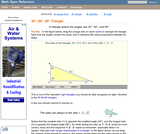Conditions of Use:
Rating

An interactive applet and associated web page that demonstrate the properties of a 30-60-90 triangle. The applet shows a right triangle that can be resized by dragging any vertex. As it is dragged, the remaining vertices change so that the triangle's angles remain 30 degrees, 60 degrees and 90 degrees The text on the page points out that the sides of a 30-60-90 triangle are always in the ratio of 1 : 2 : root 3 Applet can be enlarged to full screen size for use with a classroom projector. This resource is a component of the Math Open Reference Interactive Geometry textbook project at http://www.mathopenref.com.

Subject:
Geometry
Material Type:
Simulation
Provider:
Math Open Reference
Author:
John Page
02/16/2011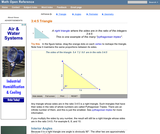Conditions of Use:
Rating

An interactive applet and associated web page that demonstrate the properties of a 3:4:5 triangle - one of the Pythagorean triples. The applet shows a right triangle that can be resized by dragging any vertex. As it is dragged, the remaining vertices change so that the triangle's side remain in the ration 3:4:5. The text on the page has an example of how the triangle can be used to measure a right angle on even large objects. Applet can be enlarged to full screen size for use with a classroom projector. This resource is a component of the Math Open Reference Interactive Geometry textbook project at http://www.mathopenref.com.

Subject:
Geometry
Material Type:
Simulation
Provider:
Math Open Reference
Author:
John Page
02/16/2011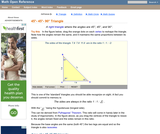Conditions of Use:
Rating

An interactive applet and associated web page that demonstrate the properties of a 45-45-90 isosceles right triangle. The applet shows a right triangle that can be resized by dragging any vertex. As it is dragged, the remaining vertices change so that the triangle's angles remain 45 degrees, 45 degrees and 90 degrees The text on the page points out that the sides of a 45-45-90 triangle are always in the ratio of 1 : 2 : root 2 Applet can be enlarged to full screen size for use with a classroom projector. This resource is a component of the Math Open Reference Interactive Geometry textbook project at http://www.mathopenref.com.

Subject:
Geometry
Material Type:
Simulation
Provider:
Math Open Reference
Author:
John Page
02/16/2011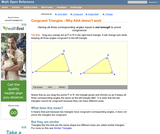Conditions of Use:
Rating

An interactive applet and associated web page that shows that angle-angle-angle (AAA) is not enough to prove congruence. The applet shows two triangles, one of which can be dragged to resize it, showing that although they have the same angles they are not the same size and thus not congruent. The web page describes all this and has links to other related pages. Applet can be enlarged to full screen size for use with a classroom projector. This resource is a component of the Math Open Reference Interactive Geometry textbook project at http://www.mathopenref.com.

Subject:
Geometry
Material Type:
Simulation
Provider:
Math Open Reference
Author:
John Page
02/16/2011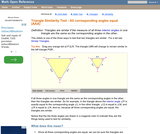Conditions of Use:
Rating

An interactive applet and associated web page showing how the AAA similarity test works. Two similar triangles are shown that can be resized by dragging. The other triangle adjusts to remain similar and the angle-angle-angle elements are highlighted to show how they are involved in this test of similarity. (all three interior angles congruent). The web page describes all this and has links to other related pages. Applet can be enlarged to full screen size for use with a classroom projector. This resource is a component of the Math Open Reference interactive geometry reference book project at http://www.mathopenref.com.

Subject:
Geometry
Material Type:
Simulation
Provider:
Math Open Reference
Author:
John Page
02/16/2011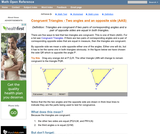Conditions of Use:
Rating

An interactive applet and associated web page that shows how triangles that have two angles and a non-included side the same must be congruent. The applet shows two triangles, one of which can be reshaped by dragging any vertex. The other changes to remain congruent to it and the two angles and non-included side are outlined in bold to show they are the same measure and are the elements being used to prove congruence. The web page describes all this and has links to other related pages. Applet can be enlarged to full screen size for use with a classroom projector. This resource is a component of the Math Open Reference Interactive Geometry textbook project at http://www.mathopenref.com.

Subject:
Geometry
Material Type:
Simulation
Provider:
Math Open Reference
Author:
John Page
02/16/2011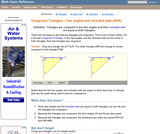Conditions of Use:
Rating

An interactive applet and associated web page that shows how triangles that have two angles and their included side the same must be congruent. The applet shows two triangles, one of which can be reshaped by dragging any vertex. The other changes to remain congruent to it and the two angles and the included side are outlined in bold to show they are the same measure and are the elements being used to prove congruence. The web page describes all this and has links to other related pages. Applet can be enlarged to full screen size for use with a classroom projector. This resource is a component of the Math Open Reference Interactive Geometry textbook project at http://www.mathopenref.com.

Subject:
Geometry
Material Type:
Simulation
Provider:
Math Open Reference
Author:
John Page
02/16/2011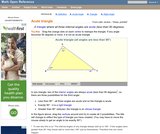Conditions of Use:
Rating

An interactive applet and associated web page that demonstrate the three types of triangle: acute, obtuse and right. The applet shows a triangle that is initially acute (all angles less then 90 degrees) which the user can reshape by dragging any vertex. There is a message changes in real time while the triangle is being dragged that tells if the triangle is an acute, right or obtuse triangle and gives the reason why. By experimenting with the triangle student can develop an intuitive sense of the difference between these three classes of triangle. Applet can be enlarged to full screen size for use with a classroom projector. This resource is a component of the Math Open Reference Interactive Geometry textbook project at http://www.mathopenref.com.

Subject:
Geometry
Material Type:
Simulation
Provider:
Math Open Reference
Author:
John Page
02/16/2011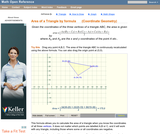Conditions of Use:
Rating

An interactive applet and associated web page that calculate the area of a triangle using the formula method in coordinate geometry. The applet has a triangle with draggable vertices. As you drag them the triangle's area is recalculated from the vertex coordinates using the formula. The grid and coordinates can be turned on and off. The area calculation can be turned off to permit class exercises and then turned back on the verify the answers. The applet can be printed as it appears on the screen to make handouts. The web page has a full description of the method for determining area using the formula method, a worked example and has links to other pages relating to coordinate geometry. Applet can be enlarged to full screen size for use with a classroom projector. This resource is a component of the Math Open Reference Interactive Geometry textbook project at http://www.mathopenref.com.

Subject:
Geometry
Material Type:
Simulation
Provider:
Math Open Reference
Author:
John Page
02/16/2011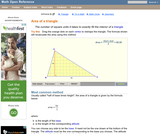Conditions of Use:
Rating

An interactive applet and associated web page that explain the area of a triangle. The applet shows a triangle that can be reshaped by dragging any vertex. As it changes, the area is continually recalculated using the 'half base times height' method. The triangle has a fixed square grid in its interior that can be used to visually estimate the area for later correlation with the calculated value. The calculation can be hidden while estimation is in progress. The text page has links to a similar page that uses Heron's Formula to compute the area. Applet can be enlarged to full screen size for use with a classroom projector. This resource is a component of the Math Open Reference Interactive Geometry textbook project at http://www.mathopenref.com.

Subject:
Geometry
Material Type:
Simulation
Provider:
Math Open Reference
Author:
John Page
02/16/2011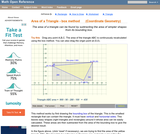Conditions of Use:
Rating

An interactive applet and associated web page that calculate the area of a triangle using the box method in coordinate geometry. The applet has a triangle with draggable vertices. As you drag them the triangle's bounding box is shown and the area recalculated by subtracting the areas of the outside triangles. The grid and coordinates can be turned on and off. The area calculation can be turned off to permit class exercises and then turned back on the verify the answers. The applet can be printed as it appears on the screen to make handouts. The web page has a full description of the method for determining area using the box method, a worked example and has links to other pages relating to coordinate geometry. Applet can be enlarged to full screen size for use with a classroom projector. This resource is a component of the Math Open Reference Interactive Geometry textbook project at http://www.mathopenref.com.

Subject:
Geometry
Material Type:
Simulation
Provider:
Math Open Reference
Author:
John Page
02/16/2011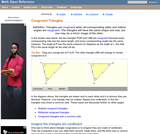Conditions of Use:
Rating

An interactive applet and associated web page that demonstrate the concept of congruent triangles. Applets show that triangles a re congruent if the are the same, rotated, or reflected. In each case the user can drag one triangle and see how another triangle changes to remain congruent to it. The web page describes all this and has links to other related pages. Applet can be enlarged to full screen size for use with a classroom projector. This resource is a component of the Math Open Reference Interactive Geometry textbook project at http://www.mathopenref.com.

Subject:
Geometry
Material Type:
Simulation
Provider:
Math Open Reference
Author:
John Page
02/16/2011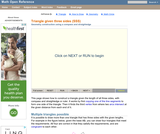Conditions of Use:
Rating

An interactive applet and associated web page that provide step-by-step animated instructions on how to construct a triangle given all three sides (SSS), using only a compass and straightedge. The animation can be run either continuously like a video, or single stepped to allow classroom discussion and thought between steps. Applet can be enlarged to full screen size for use with a classroom projector. This resource is a component of the Math Open Reference Interactive Geometry textbook project at http://www.mathopenref.com.

Subject:
Geometry
Material Type:
Simulation
Provider:
Math Open Reference
Author:
John Page
02/16/2011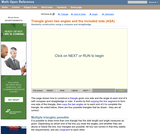Conditions of Use:
Rating

An interactive applet and associated web page that provide step-by-step animated instructions on how to construct a triangle given two angles and the included side length (ASA), using only a compass and straightedge. The animation can be run either continuously like a video, or single stepped to allow classroom discussion and thought between steps. Applet can be enlarged to full screen size for use with a classroom projector. This resource is a component of the Math Open Reference Interactive Geometry textbook project at http://www.mathopenref.com.

Subject:
Geometry
Material Type:
Simulation
Provider:
Math Open Reference
Author:
John Page
02/16/2011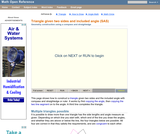Conditions of Use:
Rating

An interactive applet and associated web page that provide step-by-step animated instructions on how to construct a triangle given two sides and the included angle (SAS), using only a compass and straightedge. The animation can be run either continuously like a video, or single stepped to allow classroom discussion and thought between steps. Applet can be enlarged to full screen size for use with a classroom projector. This resource is a component of the Math Open Reference Interactive Geometry textbook project at http://www.mathopenref.com.

Subject:
Geometry
Material Type:
Simulation
Provider:
Math Open Reference
Author:
John Page
02/16/2011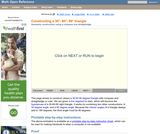Conditions of Use:
Rating

An interactive applet and associated web page that provide step-by-step animated instructions on how to construct 30-60-90 triangle with given base length using only a compass and straightedge. The animation can be run either continuously like a video, or single stepped to allow classroom discussion and thought between steps. Applet can be enlarged to full screen size for use with a classroom projector. This resource is a component of the Math Open Reference Interactive Geometry textbook project at http://www.mathopenref.com.

Subject:
Geometry
Material Type:
Simulation
Provider:
Math Open Reference
Author:
John Page
02/16/2011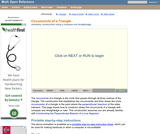Conditions of Use:
Rating

An interactive applet and associated web page that provide step-by-step instructions on how to find the circumcenter and circumcircle of a triangle using only a compass and straightedge. The method used involves constructing the perpendicular bisectors of two of the triangle's sides. The bisectors intersect at the circumcenter of the triangle. The animation can be run either continuously like a video, or single stepped to allow classroom discussion and thought between steps. Applet can be enlarged to full screen size for use with a classroom projector. This resource is a component of the Math Open Reference Interactive Geometry textbook project at http://www.mathopenref.com.

Subject:
Geometry
Material Type:
Simulation
Provider:
Math Open Reference
Author:
John Page
02/16/2011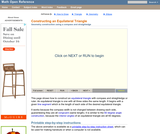Conditions of Use:
Rating

An interactive applet and associated web page that provide step-by-step instructions on how to construct an equilateral triangle with a given side length using only a compass and straightedge. The animation can be run either continuously like a video, or single stepped to allow classroom discussion and thought between steps. Applet can be enlarged to full screen size for use with a classroom projector. This resource is a component of the Math Open Reference Interactive Geometry textbook project at http://www.mathopenref.com.

Subject:
Geometry
Material Type:
Simulation
Provider:
Math Open Reference
Author:
John Page
02/16/2011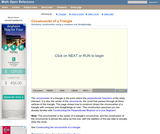Conditions of Use:
Rating

An interactive applet and associated web page that provide step-by-step animated instructions on how to construct the circumcenter of a triangle. The animation can be run either continuously like a video, or single stepped to allow classroom discussion and thought between steps. Applet can be enlarged to full screen size for use with a classroom projector. This resource is a component of the Math Open Reference Interactive Geometry textbook project at http://www.mathopenref.com.

Subject:
Geometry
Material Type:
Simulation
Provider:
Math Open Reference
Author:
John Page
02/16/2011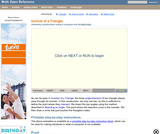Conditions of Use:
Rating

An interactive applet and associated web page that demonstrate how to construct the incenter and incircle of triangle using compass and straightedge. It starts with a given triangle, and using animation that can be run continuously or single step, shows how to first construct the incenter, then using that point, construct the incircle of the given triangle. The text on the page contains written step-by-step instructions that can be printed. Applet can be enlarged to full screen size for use with a classroom projector. This resource is a component of the Math Open Reference Interactive Geometry textbook project at http://www.mathopenref.com.

Subject:
Geometry
Material Type: